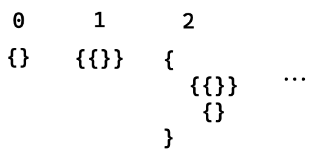# Hilbert's program

Topic | v1 | created by janarez |
Description

In mathematics, Hilbert's program, formulated by German mathematician David Hilbert in the early part of the 20th century, was a proposed solution to the foundational crisis of mathematics, when early attempts to clarify the foundations of mathematics were found to suffer from paradoxes and inconsistencies. As a solution, Hilbert proposed to ground all existing theories to a finite, complete set of axioms, and provide a proof that these axioms were consistent. Hilbert proposed that the consistency of more complicated systems, such as real analysis, could be proven in terms of simpler systems. Ultimately, the consistency of all of mathematics could be reduced to basic arithmetic. Gödel's incompleteness theorems, published in 1931, showed that Hilbert's program was unattainable for key areas of mathematics.

##### Relations

Gödel's incompleteness theorems are two theorems of mathematical logic that demonstrate the inherent...##### Resources

10.0 rating 3.0 level 8.0 clarity 4.0 background – 1 rating

In 1931, a 25-year-old Kurt Gödel wrote a proof that turned mathematics upside down. The implication...